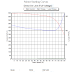The minimum start current that will start a motor is a function of the load torque and motor design

Using the simplified per phase equivalent circuit of an induction motor, one can get the following equation of electromagnetic torque:
$T_e= 3 \times \frac{I_r^2R_r}{s \omega_s}.$

$I_r = \sqrt{\frac{s \omega _s}{R_r}\times T_e}$

$T_e = J \frac{d\omega}{dt}+ T_l$
You can notice that the electromagnetic torque is proportional to moment of inertia (J) which reflects the size of the rotor or the size of the motor in general. The bigger the motor the stronger starting torque must develop to start.
$I_r = \sqrt{\frac{s \omega _s}{3\times R_r} ( J \frac{d\omega}{dt}+ T_l )}$
From the equation above the rotor current $$I_r$$ depends on $$J\frac{d\omega}{dt}$$ and the load torque $$T_l$$. Thus more load implies more rotor current.
The starting current corresponding to starting torque is the sum of inertia plus load torque, that is

$$Te= J(dw/dt)+Tl$$, where $$T_l$$ is the load torque and J(dw/dt) is the required torque to bring the rotor from standstill to the speed that makes $$Te=Tl$$.
At no-load the small current that flows through the rotor corresponds to the windage and friction torque.

Following the previous analysis, starting torque depends also on load torque $$(Tl)$$.
Maximum starting torque corresponds to full load and hence maximum starting current.

Why the Minimum Start Current of a Motor is a Function of the Load Torque and Motor DesignReviewed by Zakaria Mohammed on April 07, 2019 Rating: 5

1.2.